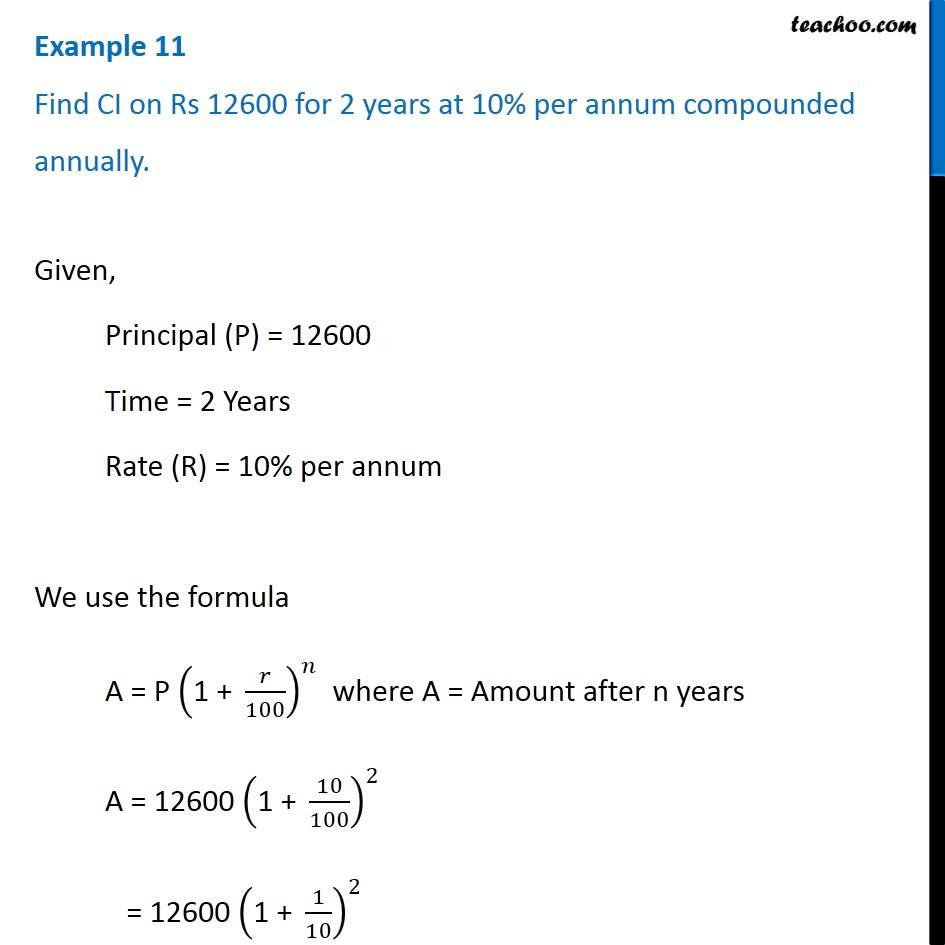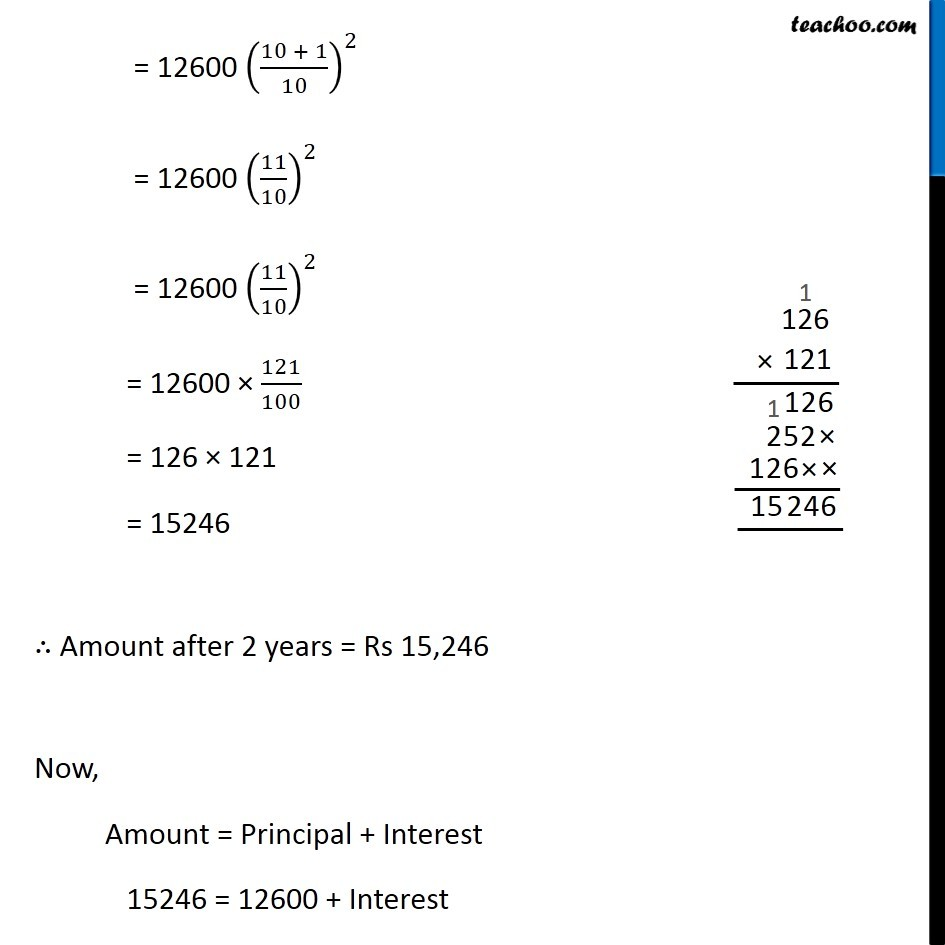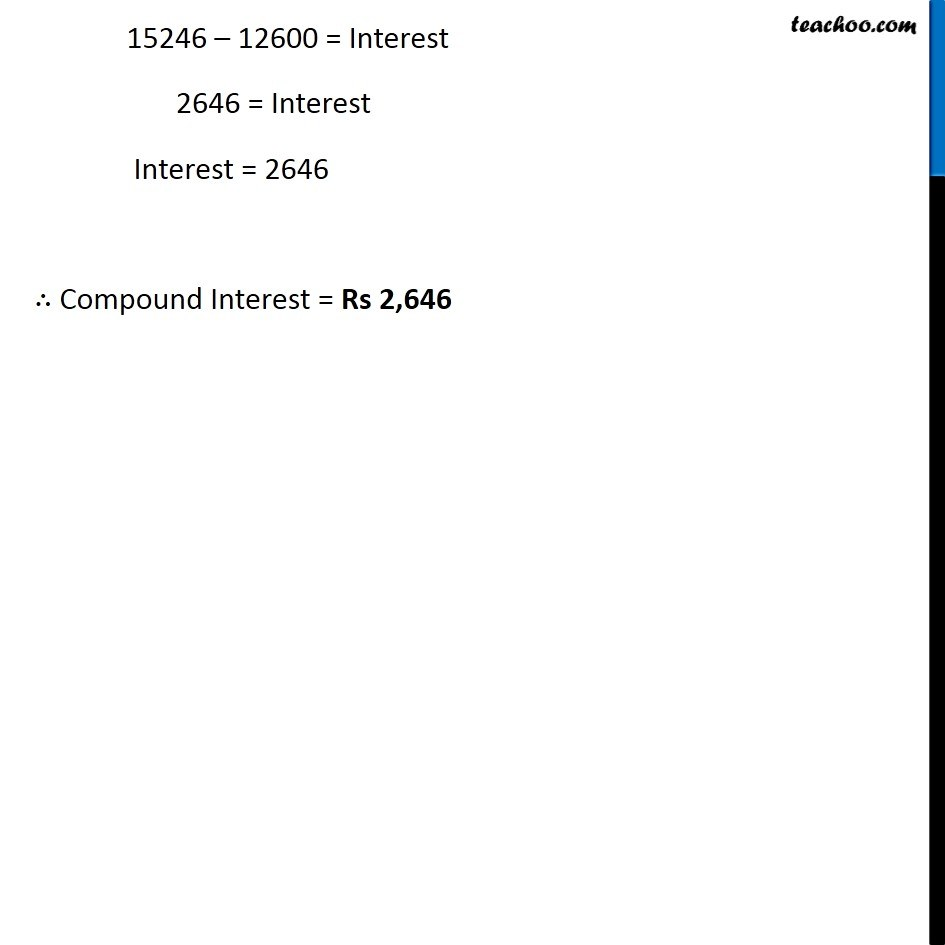Compound Interest

Chapter 8 Class 8 Comparing Quantities
Concept wiseIntroducing your new favourite teacher - Teachoo Black, at only ₹83 per month

### Transcript

Example 11 Find CI on Rs 12600 for 2 years at 10% per annum compounded annually.Given, Principal (P) = 12600 Time = 2 Years Rate (R) = 10% per annum We use the formula A = P ("1 + " 𝑟/100)^𝑛 where A = Amount after n years A = 12600 ("1 + " 10/100)^2 = 12600 ("1 + " 1/10)^2 = 12600 ((10 + 1)/10)^2 = 12600 (11/10)^2 = 12600 (11/10)^2 = 12600 × 121/100 = 126 × 121 = 15246 ∴ Amount after 2 years = Rs 15,246 Now, Amount = Principal + Interest 15246 = 12600 + Interest 15246 – 12600 = Interest 2646 = Interest Interest = 2646 ∴ Compound Interest = Rs 2,646